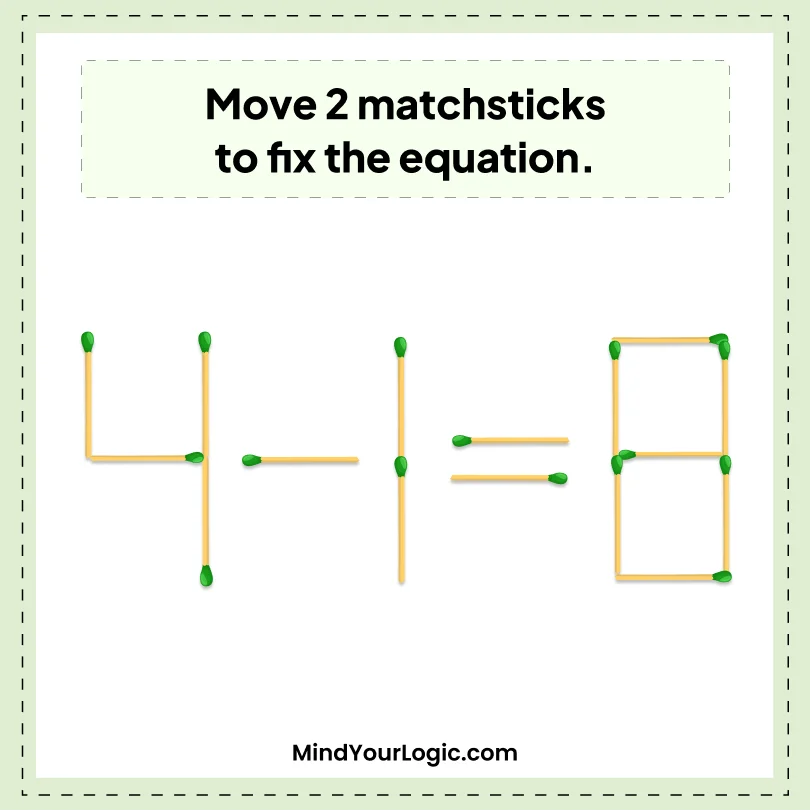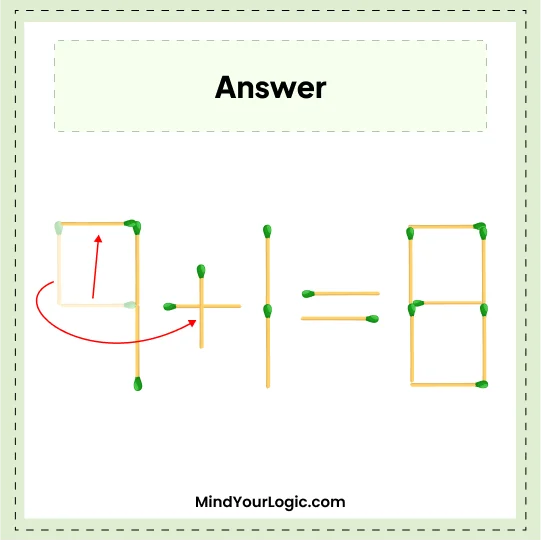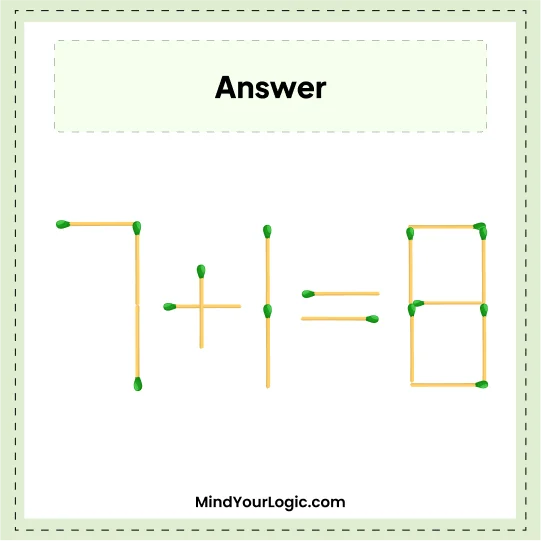# 4-1=8 - Matchstick Puzzle

###### 20.Matchstick Puzzles
`Move 2 matchsticks to fix the equation.`•

Explanation :

``` Move one stick from '4' and shift it to a - sign, which becomes +.
Next,create '4 into 7, as shown in the below image.
The correct equation will get 7+1=8.```